﻿ Experiment on the Relationship between Gravity and TemperaturePublications are Open
Access in this journal
Article Versions
Export Article
• Normal Style
• MLA Style
• APA Style
• Chicago Style
Research Article
Open Access Peer-reviewed

### Experiment on the Relationship between Gravity and Temperature

Guan Yiying, Zhang Yang , Li Huawang, Yang Fan, Guan Tianyu, Wang Dongdong, Teng Hao
International Journal of Physics. 2018, 6(4), 99-104. DOI: 10.12691/ijp-6-4-1
Published online: July 06, 2018

### Abstract

In this experiment, a number model of gravitation and temperature is established by using Cavendish twist balance to test the gravity of shot at different temperature, the relationship between gravity and temperature is analyzed quantitatively, and it is concluded that, the higher the temperature of the object, the smaller the absolute value of gravity, and vice versa. It is further deduced that the greater the velocity of the object, the smaller the absolute value of gravity, and vice versa. This experiment fully explains why the gravity is very weak under the microscopic quantum scale and high temperature in the early universe. The result of this experiment is of great significance to improve the orbit calculation precision of the spacecraft (missile) of high temperature and ultra high speed.

### 1. Introduction

Newton discovered the gravitation and established the formula of universal gravitation. This formula has been proved by numerous facts in the low-speed macro field. The current calculations of planets and missile orbits are based on Newtonian mechanics. However, there are still some problems in Newton's formula of universal gravitation, mainly in the following aspects: first, Newton's theory does not fully explain the precession of Mercury as it moves along its orbital to the perihelion. The Newtonian prediction (generated by the gravitational drag of other planets) has an error of 43 arc seconds per century compared with the actual observed precession. Second, Newton's theory predicts that the deflection of the light under the force of gravity is only half as much as the actual observation. Therefore, it can be said that Newtonian classical mechanics is a static mechanics (that is, the gravity calculation of two relatively static objects is accurate), only applicable to low speed 1 and macro field; while for the field of high speed and micro, there must be a large error in Newtonian mechanics formula, which must be corrected.

At present, many scientific research teams around the world are striving to improve the precision of Newton's gravitational constant G 2, but Liu Qi of east China university of science and technology also found that the temperature interferences with the measured value of the gravitational constant G in the experiment of measuring the gravitational constant G.

### 2. Instruments

2.1 Cavendish twist scale: Brands: KEJIAO Model: J2158 Tianjin
2.1.1. The Structure of Cavendish Twist Scale

At the ends of a light weight rod are fixed two balls (m) as the test mass. The center of the beam is suspended with a filament (commonly known as torsion wire). Two large balls near the mass of the test are usually called attractive mass as the source of interaction of gravitation to be measured. The optical lever system is consisted of the light source, the reflector that is attached to the center of the scale beam and the position detector.

2.1.2. The Working Principle of Cavendish Twist Scale

The gravitational force between the attractive mass and the test mass will add an additional torque to the torsion balance system, and the suspended wire will produce a balance torque accordingly. For the torsion balance, the deflection of balance position of torsion balance is calculated by the distance of the cursor moving on the screen of the reflector that is attached to the center of the scale beam under the effect of external torque to be measured, so as to measure the magnitude of the external force.

2.2. The Laser Source

Model:G-BJ-Y semiconductor laser Tianjin

• Figure 1. The plan schematic diagram of the principle of Cavendish twist scale
2.3. Thermometer

Brand: MATTEL, Model: MT350, Measuring range: -50°C-350°C

2.4. Electrostatic instrument

Brand: SIMCO, Model: FMX-004, Measuring range: 0-30KV

2.5. Laser range finder

Brand: SNDWAY, Model:SW-40

2.6. Two shots: the mass of each shot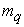: 1.5kg

2.7. Insulation cup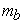: Each mass is 179g

### 3. Experimental Preparation

3.1 Determine the center of the cursor (Then no longer adjust the center of the cursor throughout the experiment)

When the shot is not placed, the mean value of the center point of the cursor swing is 35 cm on the coordinate paper, which is designated as the center of the cursor.

3.2 Measuring ambient temperature:18.4°C

3.3 Determine the corresponding distance

3.3.1 The distance between Cavendish twist scale and whiteboard L: 3.142m

3.3.2 The distance between large shot and small shot R:6.65cm

3.3.3 The cantilever of twist scale d:5.0*10-2m

3.4 After placing two shots, the cursor swing period t:9min34s

3.5 Static electricity test: The static electricity of twist scales: 0.03V, large shot A: 0.07V large shot B: 0.08V

### 4. Experimental Procedure

(1) Put two large shots into the freezer and freeze for thirty minutes.

(2) Take out the two cold shots from the freezer and place them in the same insulation cup, then they are placed at A and B, respectively.

(3) Determine the movement position of cursor every minute until the cursor is stable and no more change.

(4) Take out two large shots, measure the temperature of the two balls at that time, and then put them into boiled water for thirty minutes.

(5) Place two hot shots in two insulation cups with the same mass (the insulation cup for the hot ball is the same as that for the cold ball). and palce them at A and B, respectively.

(6) Determine the movement position of cursor every minute, until the cursor no longer changes.

(7) Take out two shots and measure the temperature of the two balls.

### 5. Experimental Data Analysis

Due to the inertial oscillation of the Cavendish scale, if only single cycle of data is used, it is obvious that there is no regularity. Therefore, we use the statistical method of periodic average, ie, the average value of the swing amplitude of one cursor is chosen for per cycle (the minimum plus maximum is divided by 2) , so this data is relatively stable and it is easy to find out the rules of data.

Through the formula: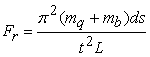(1)

Calculate the actual measured gravitational values and summarize them in Table 1:

(1) Consider that this experiment is carried out in a closed room (the doors and windows are closed, so there is no strong, continuous air flow that will have influence on the experiment. As for the occasional walks of personnel, because the walking time is short (it took 5 hours compared with this experiment),and the analytical data is the average of each vibration period, so, The possible effects of occasional walking (or other accidental factors) can also be filtered out by the use of periodic averages in the analysis data. Therefore, in this experiment, the same shot in the same place (the experiment was always in the same room) produced different gravitational values, which could only be related to the temperature of the shot.

(2) The first set of data in Table 1 shows a large “repulsion” (the absolute value of negative gravity is very large). After looking at the video, it turns out that the two insulation cups were placed at C, D position initially by experiment personnel (with cold shots) on the table. , and then immediately put them at A, B position. Because the gravitational force generated when the thermos cup is placed at C, D position exactly corresponds to the “repulsion” generated at the A, B position, therefore, the first set of data in Table 1 shows that there is a strong “repulsion” phenomenon. This strong "repulsion" phenomenon did not reappear in the second cold ball experiment.

(3) Both, the measured gravitation value increases with the rise of temperature in the cold ball experiment, and t, the gravitation value decreases with the reduce of temperature in the hot ball experimen, which are caused by the inertia of the torsion scale swing, and it is not a true reflection of the relationship between gravity and temperature.

(4) The relationship between gravity and temperature is truly reflected only by the value after the swing stabilization of the two times of cold ball experiments and the swing stabilization of the first hot ball experiment. As shown in Table 4.

• Figure 2. One dimensional graph of cursor’s mean value for each cycle in the first cold ball experiment
• Figure 3. Two dimensional graph of cursor’s mean value for each cycle in the first cold ball experiment
• Figure 4. Two dimensional graph of measured gravity for each cycle in the first cold ball experiment
• Figure 5. Two dimensional graph of measured gravity for each cycle in the first hot ball experiment
• Figure 6. Two dimensional graph of measured gravity for each cycle in the second cold ball experiment

Table 4 shows that gravitation decreases as the temperature of the object increases. On the contrary, gravity increases as the temperature of the object decreases.

### 6. Establishment of Model

According to the G Super Complex Space-time Theory, the formula of G gravitational universal gravitation is established as follows 3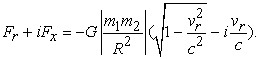(2)

The above real part is: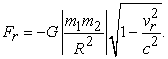(3)

The absolute value of the above formula is a constant, in which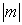is the modulus mass of the ball, and when the speed equals zero, the modulus mass equals the static mass. Therefore, static mass can be used instead of modulus mass.

Formula (3) is a correction formula for Newtonian gravitational velocity.

The imaginary part of formula (2) is: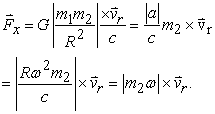(4)

The above formula is Coriolis force.

Set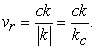(5)

k can be a temperature,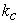is a temperature corresponding to the speed of vacuum light, which is a constant and is the upper limit of the temperature.

Bring the formula (5) into formula (2):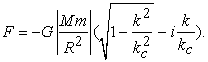(6)

The real part of formula (6) is the correction formula for Newtonian gravitational temperature, that is: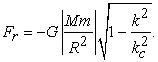(7)

### 7. Conclusion

In this experiment, the gravitation test of shot was carried out by Cavendish twist scale at different temperatures. After statistical analysis, the conclusion is as follows:

1) Newton's universal gravitational value is related to the temperature of the object.

2) The higher the temperature of the object, the smaller the absolute value of gravitation. On the contrary, the lower the temperature, the greater the absolute value of gravitation.

3) Through thermodynamics, it can be deduced that the greater the velocity of an object, the smaller the absolute value of its gravitation, conversely the lower the velocity of an object, the greater the absolute value of its gravitation.

This experiment fully explained why the gravitational force is very weak at the microscopic quantum scale and at high temperature in the early universe. The experimental result is of great significance to improve the orbital calculation precision of spacecraft (missiles) of high-temperature and ultra-high-speed.

### References

  Ni Guangjiong, Wang Yanyan, Qian Jinghua, Fang Xiaomin. Physics Change the World. Fudan University Press, Shanghai, 2016, P22. In article PubMed  Tu Liangcheng, Li Qing, Shao Chenggang, Hu Zhongkun, Luo Jun. Accurate determination of the universal gravitational constant G. Chinese Science, 2011, 41(6): 691-705. In article  Guan Yiying, Guan Tianyu. G Super Complex Space-time Theory. Heilongjiang Education Press, 2016. In article

Published with license by Science and Education Publishing, Copyright © 2018 Guan Yiying, Zhang Yang, Li Huawang, Yang Fan, Guan Tianyu, Wang Dongdong and Teng HaoThis work is licensed under a Creative Commons Attribution 4.0 International License. To view a copy of this license, visit http://creativecommons.org/licenses/by/4.0/